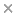BaseSpace
Correlation Engine 2.0Search sequence regions

###### filter terms:
Sizes of these terms reflect their relevance to your search.

In complex survey sampling, a fraction of a finite population is sampled. Often, the survey is conducted so that each subject in the population has a different probability of being selected into the sample. Further, many complex surveys involve stratification and clustering. For generalizability of the sample to the finite population, these features of the design are usually incorporated in the analysis. While the Wilcoxon rank sum test is commonly used to compare an ordinal variable in bivariate analyses, no simple extension of the Wilcoxon rank sum test has been proposed for complex survey data. With multinomial sampling of independent subjects, the Wilcoxon rank-sum test statistic equals the score test statistic for the group effect from a proportional odds cumulative logistic regression model for an ordinal outcome. Using this regression framework, for complex survey data, we formulate a similar proportional odds cumulative logistic regression model for the ordinal variable, and use an estimating equations score statistic for no group effect as an extension of the Wilcoxon test. The proposed method is applied to a complex survey designed to produce national estimates of the health care use, expenditures, sources of payment, and insurance coverage.

### Citation

Sundar Natarajan, Stuart R Lipsitz, Garrett M Fitzmaurice, Debajyoti Sinha, Joseph G Ibrahim, Jennifer Haas, Walid Gellad. An extension of the Wilcoxon Rank-Sum test for complex sample survey data. Journal of the Royal Statistical Society. Series C, Applied statistics. 2012 Aug;61(4):653-664

PMID: 23913985

View Full Text